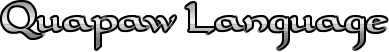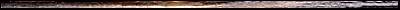where, which direction

 ha-ki-wa-de-de (hákiwadede) how do you get over there, which road, how do you go Speaker - Maude Supernaw

______________________________________________________________________________

ha-ki-de-de (hákidede)

where, which direction (RR-Quapaw)

______________________________________________________________________________

Dhegiha Language Family Comparison

• ha-ki-de-de (hákidede) - where, which direction (RR-Quapaw)
• ha-ki-de-de-tʰa (hákidedétʰą) - where from, from whatever place (RR-Quapaw)

______________________________________________________________________________

• ha-ki (hakí) - where (RR-Quapaw)
• ha-ge-i (ha-ge-i) - where? (LH-Quapaw)
• ho-wa-gi (hó-wa-gi) - where (FL-Osage)
• ho-waiⁿ-ki (howáįki) - where, wherein, where at, where to, be where, wherever, be wherever (CQ-Osage)
• ho-wa-ge-ji (hówagèji) - where, to what place, at what place (Kanza)